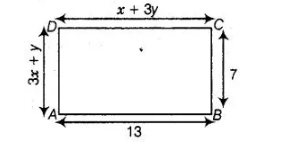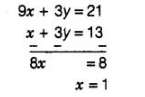# Find the values of x and y in

Question:

Find the values of x and y in the following rectangleSolution:

By property of rectangle,

Lengths are equal, i.e., $C D=A B$

$\Rightarrow$ $x+3 y=13$ $\ldots$ (i)

Breadth are equal, i.e., $A D=B C$

$\Rightarrow$ $3 x+y=7$ ...(ii)

On multiplying Eq. (ii) by 3 and then subtracting Eq. (i), we getOn putting $x=1$ in Eq. (i), we get

$3 y=12 \Rightarrow y=4$

Hence, the required values of $x$ and $y$ are 1 and 4 , respectively.